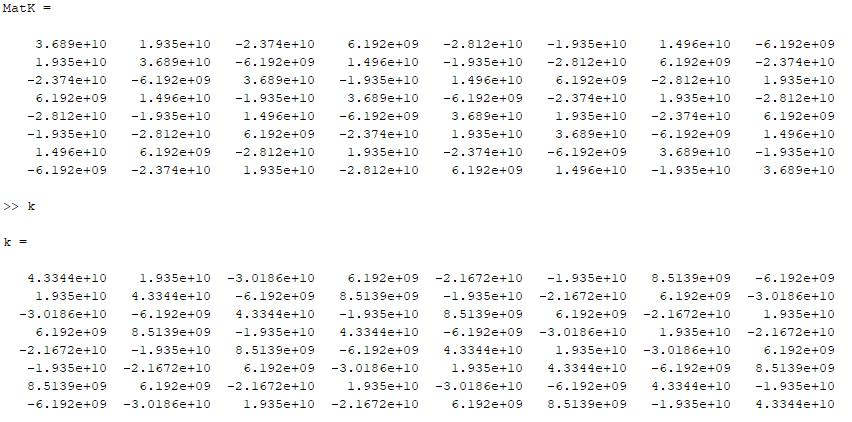## General Mechanical

Topics relate to Mechanical Enterprise, Motion, Additive Print and more

•ericzhng
Subscriber

I used a set of simple APDL commands (attached below) trying to get the local stiffness matrix (8 X. The results I got is somehow different from what I compute by hand. This is for a 2D plane element (1 element), no constraints, applied with a zero force. This way we can get the full local stiffness matrix.

Also when I tried to derive mass matrix, which I have to specify a transient analysis, the local stiffness matrix changes to a 25 X 25 size matrix. And still I couldn't output the mass matrix, not sure why.

•John Doyle
Ansys Employee

Try using the "EMATWRITE,yes" before the SOLVE.

and then after the solve, add the following:

/POST1

*DMAT, stiffness, D, Import, EMAT,plane2d.emat, STIFF, 1

*PRINT,stiffness,stiffness_182.txt

*DMAT, mass, D, Import, EMAT,plane2d.emat, MASS, 1

*PRINT,mass,mass_182.txt

•ericzhng
Subscriber

Thanks Doyle, That is great help! I was wondering why I can't extract mass matrix.

But same issue exists that the stiffness matrix I obtained from ANSYS and the one I computed are different. I double checked my formulation of 2d element, it should be correct.The matK is what I imported from ANSYS, the other k is what I computed. Could you give me some hints on this, please?

Thank you!

•ericzhng
Subscriber

Sorry, I forgot to reply to you in last post.

•emix
Subscriber

I know this post is old, but in case anybody is still interested, the difference in stiffness matrix between plane182 element and the standard Q4 elements is that the first has enhanced strain formulations to prevent shear locking.

To deactivate this function just use the following:

KEYOPT,1,1,0

By setting KEYOPT(1)=0, the stiffness matrix obtained from ANSYS would be the same as the one computed.